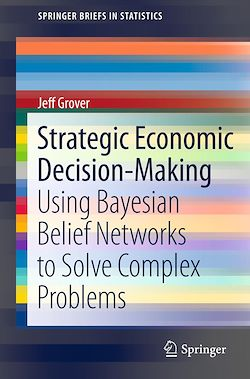# Strategic Economic Decision-Making

-English
116 Pages

Description

Strategic Economic Decision-Making: Using Bayesian Belief Networks to Solve Complex Problems is a quick primer on the topic that introduces readers to the basic complexities and nuances associated with learning Bayes’ theory and inverse probability for the first time. This brief is meant for non-statisticians who are unfamiliar with Bayes’ theorem, walking them through the theoretical phases of set and sample set selection, the axioms of probability, probability theory as it pertains to Bayes’ theorem, and posterior probabilities. All of these concepts are explained as they appear in the methodology of fitting a Bayes’ model, and upon completion of the text readers will be able to mathematically determine posterior probabilities of multiple independent nodes across any system available for study.  Very little has been published in the area of discrete Bayes’ theory, and this brief will appeal to non-statisticians conducting research in the fields of engineering, computing, life sciences, and social sciences.

Subjects

##### Statistics

Informations